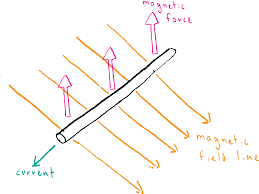# Magnetic force on a wire

## Homework Statement

A wire with a current of I (flowing into the screen) moves down on a slope.
What is the direction of the magnetic force acting on it?

## The Attempt at a Solution

I have no Idea why the answer isn't horizontal, but rather opposing its velocity direction.

#### Attachments

•ללא שם.png
8.7 KB · Views: 449

BvU
Homework Helper
Says who ?
And: What exactly is your idea of the force ?
"request": Use the template in the way it is meant to be used...

Says who ?
And: What exactly is your idea of the force ?
"request": Use the template in the way it is meant to be used...
Says the answer in the book.
This is the EXACT setupAnd in three different place I saw something different.

#### Attachments

haruspex
Homework Helper
Gold Member
Says the answer in the book.
This is the EXACT setup View attachment 227008
And in three different place I saw something different.
In your original diagram you had the field vertical, but now you show force as vertical and field acting in the direction of v. Have you mislabelled the second diagram?
Also, the question as stated makes no reference to a field in the text. That only appears in the diagram. Did you quote the whole text?

In your original diagram you had the field vertical, but now you show force as vertical and field acting in the direction of v. Have you mislabelled the second diagram?
Also, the question as stated makes no reference to a field in the text. That only appears in the diagram. Did you quote the whole text?
I apologize, this picture isn't the same.
The magnetic field is indeed vertical, as shown before. This is just another angle to look at it (This picture doesn't have anything to do with the problem, just the angle).

haruspex
Homework Helper
Gold Member
This picture doesn't have anything to do with the problem, just the angle
So what, exactly, is the answer given in the book?

So what, exactly, is the answer given in the book?
It says the force is opposing the velocity.

haruspex
Homework Helper
Gold Member
It says the force is opposing the velocity.
That is a bit vague. It does not necessarily mean that it acts antiparallel to the velocity, merely that it is in a direction that resists the movement.

TSny
Homework Helper
Gold Member

## Homework Statement

A wire with a current of I (flowing into the screen) moves down on a slope.
What is the direction of the magnetic force acting on it?
Please make sure you have stated the problem exactly as given, word-for-word. Sometimes, the reason a student is having difficulty is that they have misinterpreted the wording of the problem.

Also, you attached a figure in your first post. Is this a figure that was provided with the statement of the problem, or is this a figure that you constructed yourself?

lekh2003
Gold Member
That is a bit vague. It does not necessarily mean that it acts antiparallel to the velocity, merely that it is in a direction that resists the movement.
I think what the textbook means to say is that the force is in the exact opposite direction of the current. I don't think a simple question from an assumable simple textbook would say otherwise.

For the OP, I simple don't seem to understand what you are trying to say. Start from the beginning, quote the text of the problem, quote the answer. Only show us what is in the textbook. Then, completely separately, give us your opinion and what you think is happening.

haruspex
Homework Helper
Gold Member
what the textbook means to say is that the force is in the exact opposite direction of the current.
Of the current? You mean, of the movement, perhaps? That is how Eitan is reading it, but that answer would be wrong.

lekh2003
Gold Member
Of the current? You mean, of the movement, perhaps? That is how Eitan is reading it, but that answer would be wrong.
Ah, yes the movement. Opposite the movement. But OP simply needs to make the problem a little more clear.

haruspex
Homework Helper
Gold Member
Opposite the movement.
But it would not be directly opposite to the movement, would it?

lekh2003
Gold Member
But it would not be directly opposite to the movement, would it?
Okay, so if we use the right hand rule with the velocity downwards and to the right, and the magnetic field upwards, then the force moves with the current. So, it is parallel, but not opposite. This is confusing me as well now, I might as well wait for the OP to respond and then continue.

haruspex
Homework Helper
Gold Member
the force moves with the current.
Not sure what you mean by that. There will be an induced current. It is unclear to me whether the current stated in the question is this induced current or additional to it, but whatever...
The force that results should be normal to the field and to the current, so horizontal.

## Homework Statement

A wire with a current of I (flowing into the screen) moves down on a slope.
What is the direction of the magnetic force acting on it?

## The Attempt at a Solution

I have no Idea why the answer isn't horizontal, but rather opposing its velocity direction.
Try googling Lenz's law.

haruspex